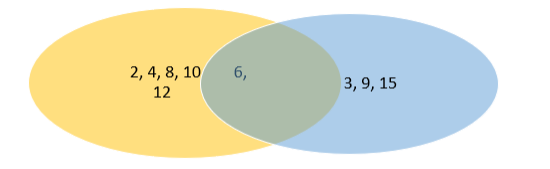# Using Venn diagrams, show that

Question:

Using Venn diagrams, show that $(A-B), A \cap B)$ and $(B-A)$ are disjoint sets,taking $A=\{2,4,6,8,10,12\}$ and $B=\{3,6,9,12,15,$,$} .$

Solution:A - B is denoted by the yellow region only

B - A is denoted by the blue region only

AB is denoted by the common region (blue +yellow)

There is no intersection between these three regions

Hence the three sets are disjoint sets.